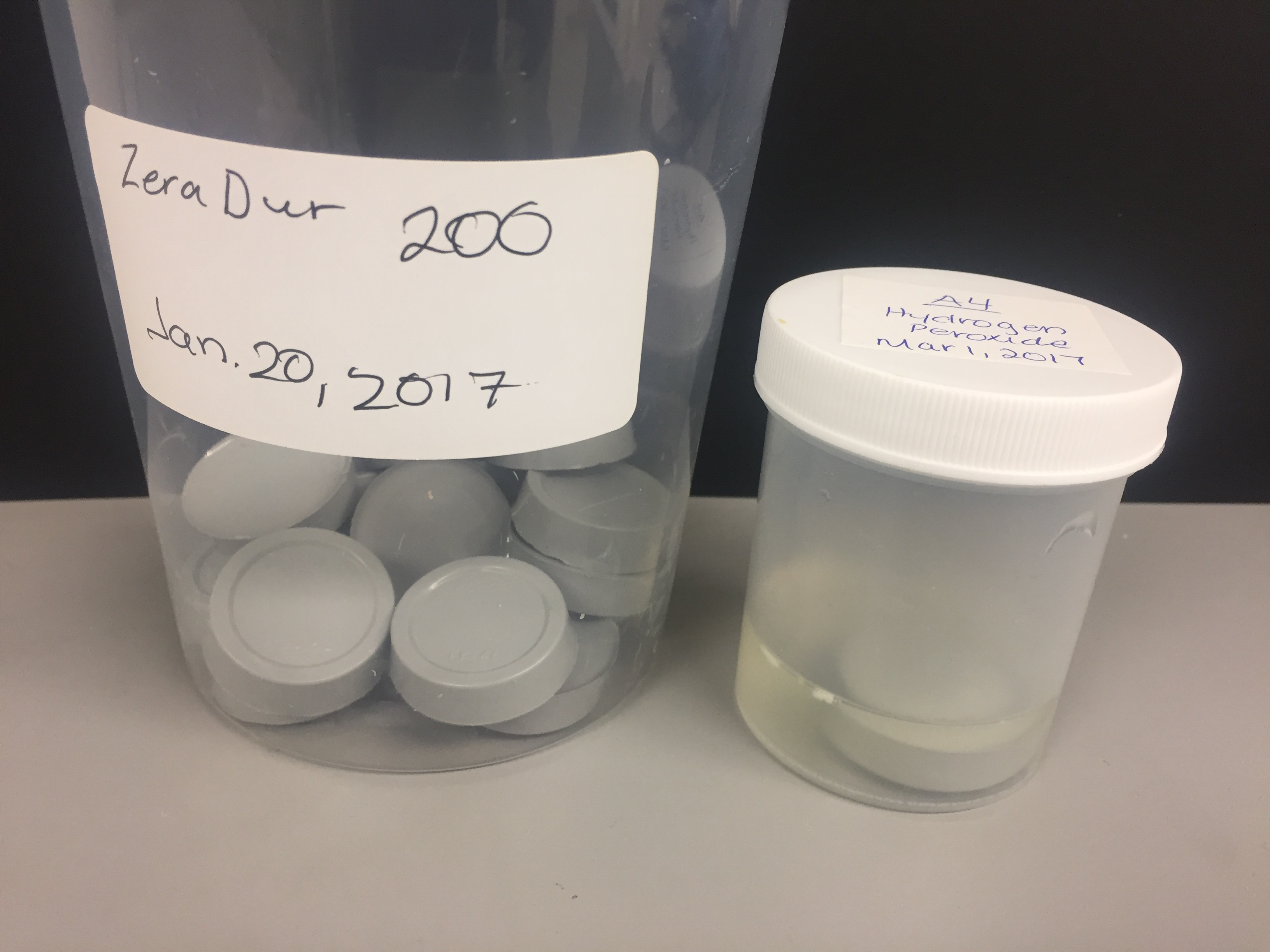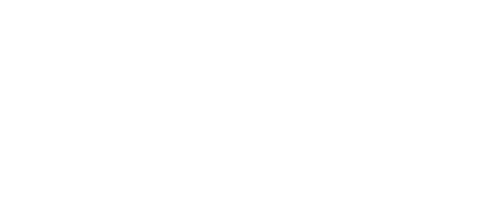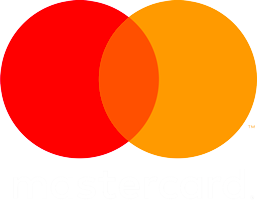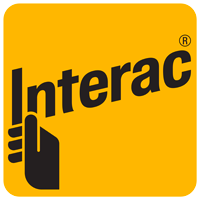# Chemical Resistance Charts for Flooring and Coatings

ASTM C722 Chemical Resistance Test: 7 Days Full Immersion @ 23°C/50% RH (7 Days Full Cure)

ACIDS

 Chemicals Conc. % ZeraDur 200 ZeraDur N300 CR ZeraKlear CR ZeraClad 100 Acetic Acid 10% R R* R L 20% R R* R NR 40% S R* S NR glac. NR NR NR Battery Acid R R R R Benzoic Acid 10% R R R R Boric Acid 10% R R R R Citric Acid 25% R R R R 50% R R R R Chromic Acid 10% R R R R 25% R R R R 60% R R R R Formic Acid 10% R R* S R 20% R R* S NR 50% R R* NR NR Flourosilicic Acid 10% R R R R 35% R R R R Glycolic Acid 20% R R S NR 40% S S NR NR 98% S S NR NR Hydrochloric Acid 20% R* R R R 36% R* R R R Lactic Acid 20% S R R NR 40% S R R NR 85% S R R L Nitric Acid 20% R(D)* R(D) R(D) R(D) 40% R(D)* R(D) R(D) R 60% R(D)* R(D) S R 70% R(D)* R(D) S NR Sulphuric Acid 10% R* R R R 40% R R R R 70% R* R(D) R(D) L 98% NR R* NR NR Phosphoric Acid 10% R* R R R 50% R* R R L 75% R* R R L Tartaric Acid 20% R R R R 50% R R R R

RATING LEGEND

 R - Recommended NR - Not Recommended S - Splash and Spillage (D) - Discolouration R* - Best Recommendation

Note: some discoloration might not be acceptable for customer in certain applications. Please check before making recommendation.

SOLVENTS

 Chemicals ZeraDur 200 ZeraDur N300 CR ZeraKlear CR ZeraClad 100 Acetate, PM R R R R Acetone NR NR NR NR Benzyl Alcohol S R* R S Butanol R R R R n.Butyl Acetate R R R NR Butyl Carbitol R R R S Cyclohexanol R R R R Dibasic Ester R R R R D-Limonene R R R R Ethanol R R R R Furfural Alcohol NR NR NR Isopropanol R R R R Methanol R* NR NR Methyl Ethyl ketone (MEK) NR R* R* NR Methyl Isobutyl Ketone (MIBK) R* R R R Mineral Spirits R R R R Naphtha, H.F. R R R R Oxol 100 R R R R Varsol R R R R Xylene R R R NR

RATING LEGEND

 R - Recommended NR - Not Recommended S - Splash and Spillage (D) - Discolouration R* - Best Recommendation

Note: some discoloration might not be acceptable for customer in certain applications. Please check before making recommendation.

BASES

 Chemicals ZeraDur 200 ZeraDur N300 CR ZeraKlear CR ZeraClad 100 Ammonium Benzoate R R R R Ammonium Hydroxide R R R R Potassium Hydroxide R R R R Potassium Hydroxide R R R R Sodium Chloride R R R R Sodium Hydroxide R R R R Sodium Hypochlorite R S R S Trisodium Phosphate R R R R Zinc Oxide Solution R R R R Zinc Sulphate R R R R Calcium Chloride R R R R Sodium Sulphide R R R R

RATING LEGEND

 R - Recommended NR - Not Recommended S - Splash and Spillage (D) - Discolouration R* - Best Recommendation

Note: some discoloration might not be acceptable for customer in certain applications. Please check before making recommendation.

PETROLEUM PRODUCTS

 Chemicals ZeraDur 200 ZeraDur N300 CR ZeraKlear CR ZeraClad 100 Brake Fluid R R R R Diesel Fuel R R R R Engine Oil (10 W30) R R R R Esso Turbo Oil R R R R Gasoline R R R R Hydraulic Fluid R R R R Jet Fuel R R R R Skydrol R R R R Brine R R R R L-602 R R R R Transformer Oil R R R R Transmission Fluid R R R R Turbo Fuel B R R R R

RATING LEGEND

 R - Recommended NR - Not Recommended S - Splash and Spillage (D) - Discolouration R* - Best Recommendation

Note: some discoloration might not be acceptable for customer in certain applications. Please check before making recommendation

CHEMICALS

 Chemicals ZeraDur 200 ZeraDur N300 CR ZeraKlear CR ZeraClad 100 Benzyl Chloride S R S S Chlorine R R R R Diamines R R R R Ethyl Morpholine R R R R Formaldehyde R R R R Glycerin R R R R Hydrazine Hydrate R R R S Hydogen Peroxide R R R S Pyridine R R R R Styrene R R R R

RATING LEGEND

 R - Recommended NR - Not Recommended S - Splash and Spillage (D) - Discolouration R* - Best Recommendation

Note: some discoloration might not be acceptable for customer in certain applications. Please check before making recommendation.

FOOD & MISC.

 Chemicals ZeraDur 200 ZeraDur N300 CR ZeraKlear CR ZeraClad 100 Beer R R R R Blood R R RR R Coffee R R R R Coke R R R R Cooking Oil R R R R Honey R R R R Hot Animal Fat R R R R Javex R R R R Ketchup R R R R Lard R R R R Margarine R R R R Milk R R R R Mustard R R R R Pine Oil R R R R Tea R R R R Acidic Waste Water @180F NR R* NR NR Urine (Animal) R R R R

RATING LEGEND
 R - Recommended NR - Not Recommended S - Splash and Spillage (D) - Discolouration R* - Best Recommendation

Note: some discoloration might not be acceptable for customer in certain applications. Please check before making recommendation.

CHEMICAL RESISTANCE OF WATERBORNE COATINGS

ASTM D 1308 – Covered Spot Test, 24hrs @ 23C

 Chemical ZeraTuf W-104 ZeraTuf W-104 with Crosslinker ZeraTuf W-104 Grey Baking Soda No Effect No Effect No Effect Sugar No Effect No Effect No Effect Sulphuric Acid (10%) No Effect No Effect Stained Chlorine No Effect No Effect No Effect Lactic Acid (10%) Stained Stained Stained Colorax (50%) No Effect No Effect No Effect Windex No Effect No Effect No Effect Coffee Stained Stained Stained Ethanol (50%) Stained Stained No Effect CLR No Effect No Effect Stained White Wine No Effect No Effect No Effect Red Wine Stained Stained Stained Vinegar No Effect No Effect No Effect Tea No Effect No Effect Stained Milk No Effect No Effect No Effect Ketchup No Effect No Effect Stained Mustard Stained Stained Stained Cooking Oil No Effect No Effect No Effect Leather Cleaner No Effect No Effect No Effect Windsheild Washer Fluid No Effect No Effect No Effect

NOTE: The information contained herein is given in good faith to be used as a guide for product selection. Since operating conditions are beyond our control, customers must ensure that the product is suitable for the purpose in which it is intended. All information contained in this Chemical Resistance Chart is to be used as a guide and is subject to change without notice.

We accept the following payment methods for Zeraus orders

•••